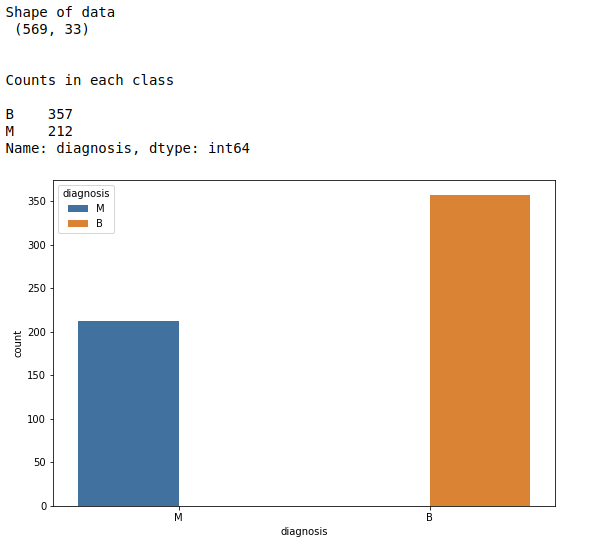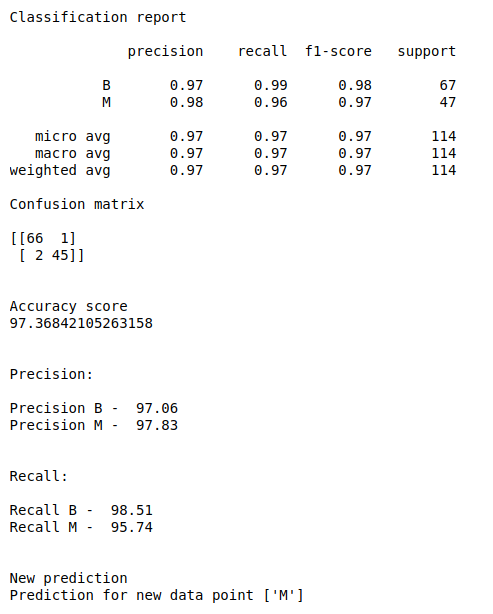• #5, First Floor, 4th Street Dr. Subbarayan Nagar Kodambakkam, Chennai-600 024 Landmark : Samiyar Madam
• pro@slogix.in
• +91- 81240 01111

### How to predict breast cancer using Support Vector Machine in python?

###### Description

To predict whether the patient having breast cancer or not using machine learning in python.

#### Input

Breast cancer data set. (Kaggle).

#### Output

Classification report, accuracy score and confusion matrix.

New prediction for new data point.

###### Process

Import libraries.

Load the data set.

Fix X and y variables.

Split the data into train and test.

Build the MLP model.

Fit the train data to the model.

Predict the test data.

Evaluate the model.

Print the results.

###### Sample Code

#import libraries
import pandas as pd
import numpy as np
import matplotlib.pyplot as plt
import seaborn as sns
from sklearn.preprocessing import StandardScaler
from sklearn.model_selection import train_test_split
from sklearn.svm import SVC
from sklearn.metrics import classification_report, confusion_matrix, accuracy_score

#check missing values
print(“Checking missing values\n”)
print(data.isnull().sum())

#make it as a data frame
df = pd.DataFrame(data)
print(“\n”)

#print data shape
print(“Shape of data\n”,df.shape)

#counts in each class
print(“\n”)
print(“Counts in each class\n”)
count = df[‘diagnosis’].value_counts()
print(count)

#Count plot for target
plt.rcParams[“figure.figsize”] = [9,6]
sns.countplot(x=’diagnosis’,hue=”diagnosis”, data=df)
plt.show()

#Define X and y variable
X = df.iloc[:,2:32]
y = df.iloc[:,1]

#scale the input data
sc = StandardScaler()
X = sc.fit_transform(X)

#Split train and test data
X_train, X_test, y_train, y_test = train_test_split(X, y, test_size=0.2, random_state=0)

#training set and testing set
print(“\n”)
print(“Training data set\n”,X_train,”\n”,y_train)
print(“\n”)
print(“Testing data set\n”,X_test)

#Build the classifier
classifier = SVC(kernel=’linear’,probability=True)

#train the classifier
classifier.fit(X_train,y_train)

#predict the test data
y_pred = classifier.predict(X_test)

#Evaluate the model
print(“\n”)
print(“Classification report\n”)
print(classification_report(y_test, y_pred))
print(“Confusion matrix\n”)
print(confusion_matrix(y_test, y_pred))
print(“\n”)
print(“Accuracy score”)
print(accuracy_score(y_test, y_pred)*100)
print(“\n”)

#Precision calulation from scratch
cm = confusion_matrix(y_test, y_pred)
print(“Precision:\n”)
def precision(cm):
p = (cm/((cm)+(cm)))
if (str(p) == ‘nan’):
print(“Precision B – “,”0.00”)
else:
print(“Precision B – “,round(p*100,2))
precision(cm)

def precision1(cm):
p1 = (cm/((cm)+(cm)))
if (str(p1) == ‘nan’):
print(“Precision M – “,”0.00”)
else:
print(“Precision M – “,round(p1*100,2))
precision1(cm)

#recall calculation
print(“\n”)
print(“Recall:\n”)
def recall(cm):
p = (cm/((cm)+(cm)))
if (str(p) == ‘nan’):
print(“Recall B – “,”0.00”)
else:
print(“Recall B – “,round(p*100,2))
recall(cm)

def recall1(cm):
p1 = (cm/((cm)+(cm)))
if (str(p1) == ‘nan’):
print(“Recall M – “,”0.00”)
else:
print(“Recall M – “,round(p1*100,2))
recall1(cm)

#predict new comment
print(“\n”)
print(“New prediction”)
new = [[15.34,14.26,102.5,704.4,0.1073,0.2135,0.2077,0.09756,0.2521,0.07032,0.4388,0.7096,3.384,44.91,0.006789,
0.05328,0.06446,0.02252,0.03672,0.004394,18.07,19.08,125.1,980.9,0.139,0.5954,0.6305,0.2393,0.4667,0.09946]]
new_pred = classifier.predict(new)
print(“Prediction for new data point”,new_pred)

###### Screenshots